Home > MC2 > Chapter 11 > Lesson 11.3.3 > Problem11-113

11-113.
1. Simplify each expression. Homework Help ✎

1.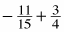2.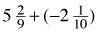3.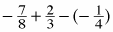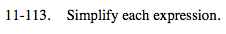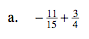Find a common denominator.

$- \frac{44}{60} + \frac{45}{60}$

$\frac{1}{60}$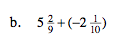Use the associative property of addition.

$(5-2) + ( \frac{2}{9} - \frac{1}{10} )$

$3 \frac{11}{90}$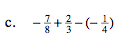See (a).